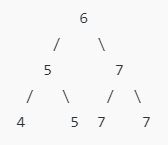New update is available. Click here to update.

# BALLOONS GAME

Posted: 23 Mar, 2021
Difficulty: Easy

## PROBLEM STATEMENT

#### Your task is to write a code that can find the element with a maximum frequency in the given binary search tree you will be provided with the root of the tree.

##### Example:
``````Consider the following binary search tree so the ‘7’ is the element with the highest repeated frequency i.e ‘3’ so ‘7’ is our answer.
``````#### Note :

``````If there is more than one element with the same maximum frequency you have to return a minimum of them.
``````
##### Input Format:
``````The first line contains an integer  ‘T' which denotes the number of test cases or queries to be run. Then the test cases follow.

The first line of each test case contains the elements of the tree in the level order form separated by a single space.

If any node does not have a left or right child, take -1 in its place. Refer to the example below.

Example:

Elements are in the level order form. The input consists of values of nodes separated by a single space in a single line. In case a node is null, we take -1 in its place.

For example, the input for the tree depicted in the below image would be :
````````````1
2 3
4 -1 5 6
-1 7 -1 -1 -1 -1
-1 -1

Explanation :
Level 1 :
The root node of the tree is 1

Level 2 :
Left child of 1 = 2
Right child of 1 = 3

Level 3 :
Left child of 2 = 4
Right child of 2 = null (-1)
Left child of 3 = 5
Right child of 3 = 6

Level 4 :
Left child of 4 = null (-1)
Right child of 4 = 7
Left child of 5 = null (-1)
Right child of 5 = null (-1)
Left child of 6 = null (-1)
Right child of 6 = null (-1)

Level 5 :
Left child of 7 = null (-1)
Right child of 7 = null (-1)

The first not-null node (of the previous level) is treated as the parent of the first two nodes of the current level. The second not-null node (of the previous level) is treated as the parent node for the next two nodes of the current level and so on.

The input ends when all nodes at the last level are null (-1).
``````
##### Note :
``````The above format was just to provide clarity on how the input is formed for a given tree.

The sequence will be put together in a single line separated by a single space. Hence, for the above-depicted tree, the input will be given as:

1 2 3 -1 5 6 7 -1 -1 -1 -1
``````
##### Output Format :
``````For each test case, print the node with maximum repeated frequency.

Print output of each test case in a separate line.
``````
##### Note:
``````You are not required to print anything explicitly. It has already been taken care of. Just implement the function.
``````
##### Constraints:
``````1 <= T <= 100
1 <= N <= 3000
0 <= DATA <= 10^5

Time Limit: 1 sec
``````
SIMILAR PROBLEMS

Median Marks

Posted: 11 Aug, 2022
Difficulty: Hard

Beautiful Distribution

Posted: 2 Sep, 2022
Difficulty: Moderate

Max Prefix

Posted: 31 Oct, 2022
Difficulty: Easy

Merge Two Sorted Arrays Without Extra Space

Posted: 19 Nov, 2022
Difficulty: Moderate

Sort 0s, 1s, 2s

Posted: 24 Dec, 2022
Difficulty: Easy